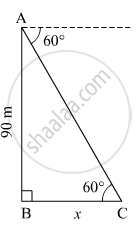Share

# From the Top of a Lighthouse, an Observer Looking at a Ship Makes Angle of Depression of 60°. If the Height of the Lighthouse is 90 Metre, Then Find How Far the Ship is from the Lighthouse. - Geometry

ConceptHeights and Distances

#### Question

From the top of a lighthouse, an observer looking at a ship makes angle of depression of 60°. If the height of the lighthouse is 90 metre, then find how far the ship is from the lighthouse.

$\left( \sqrt{3} = 1 . 73 \right)$

#### Solution

Let AB be the lighthouse and C be the position of the ship from the lighthouse.
Suppose the distance of the ship from the lighthouse be x m.In right ∆ABC,
$\tan60^\circ = \frac{AB}{BC}$
$\Rightarrow \sqrt{3} = \frac{90}{x}$
$\Rightarrow x = \frac{90}{\sqrt{3}} = 30\sqrt{3}$
$\Rightarrow x = 30 \times 1 . 73 = 51 . 9 m$

Thus, the ship is 51.9 m away from the lighthouse.

Is there an error in this question or solution?

#### Video TutorialsVIEW ALL 

Solution From the Top of a Lighthouse, an Observer Looking at a Ship Makes Angle of Depression of 60°. If the Height of the Lighthouse is 90 Metre, Then Find How Far the Ship is from the Lighthouse. Concept: Heights and Distances.
S